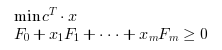Scilab Home page | Wiki | Bug tracker | Forge | Mailing list archives | ATOMS | File exchange
Change language to: English - Français - Português - 日本語 -

See the recommended documentation of this function

# semidef

Solve semidefinite problems.

### Calling Sequence

```x=semidef(x0,Z0,F,blocksizes,c,options)
[x,Z]=semidef(...)
[x,Z,ul]=semidef(...)
[x,Z,ul,info]=semidef(...)```

### Arguments

x0

m-by-1 real column vector (must be strictly primal feasible, see below)

Z0

L-by-1 real vector (compressed form of a strictly feasible dual matrix, see below)

F

L-by-(m+1) real matrix

blocksizes

p-by-2 integer matrix (sizes of the blocks) defining the dimensions of the (square) diagonal blocks `size(Fi(j)=blocksizes(j) j=1,...,m+1`.

c

m-by-1 real vector

options

a 1-by-5 matrix of doubles `[nu,abstol,reltol,tv,maxiters]`

ul

a 1-by-2 matrix of doubles.

### Description

`semidef` solves the semidefinite program:and its dual:exploiting block structure in the matrices `F_i`. Here, `Tr` is the trace operator, i.e. the sum of the diagonal entries of the matrix.

The problem data are the vector `c` and `m+1` block-diagonal symmetric matrices `F0`, `F1`, ..., `Fm`. Moreover, we assume that the matrices `Fi` have `L` diagonal blocks.

The `Fi's` matrices are stored columnwise in `F` in compressed format: if `F_i^j`, i=0,..,m, j=1,...,L denote the jth (symmetric) diagonal block of `F_i`, thenwhere, for each symmetric block `M`, the vector `cmp(M)` is the compressed representation of `M`, that is `[M(1,1);M(1,2);...;M(1,n);M(2,2);M(2,3);...;M(2,n);...;M(n,n)]`, obtained by scanning rowwise the upper triangular part of `M`.

For example, the matrixis stored as

```            [1; 2; 3; 4; 5; 6; 7; 8; 9; 10]
```

with `blocksizes=[2,3,1]`.

In order to create the matrix `F`, the user can combine the `list2vec` and `pack` function, as described in the example below.

`blocksizes` gives the size of block `j`, ie, `size(F_i^j)=blocksizes(j)`.

Z is a block diagonal matrix with L blocks ```Z^0, ..., Z^{L-1}``` .`Z^j` has size ```blocksizes[j] times blocksizes[j]``` .Every block is stored using packed storage of the lower triangular part.

The 1-by-2 matrix of doubles `ul` contains the primal objective value `c'*x` and the dual objective value `-trace(F_0*Z`).

The entries of `options` are respectively:

• `nu`: a real parameter which controls the rate of convergence.

• `abstol`: absolute tolerance. The absolute tolerance cannot be lower than 1.0e-8, that is, the absolute tolerance used in the algorithm is the maximum of the user-defined tolerance and the constant tolerance 1.0e-8.

• `reltol`: relative tolerance (has a special meaning when negative).

• `tv`: the target value, only referenced if `reltol < 0`.

• `maxiters`: the maximum number of iterations, a positive integer value.

On output, the `info` variable contains the status of the execution.

• `info=1` if maxiters exceeded,

• `info=2` if absolute accuracy is reached,

• `info=3` if relative accuracy is reached,

• `info=4` if target value is reached,

• `info=5` if target value is not achievable;

• negative values indicate errors.

Convergence criterion is based on the following conditions that is, the algorithm stops if one of the following conditions is true:

• maxiters is exceeded

• duality gap is less than abstol

• primal and dual objective are both positive and duality gap is less than (`reltol` * dual objective) or primal and dual objective are both negative and duality gap is less than (`reltol` * minus the primal objective)

• reltol is negative and primal objective is less than tv or dual objective is greater than `tv`.

### Examples

```// 1. Define the initial guess
x0=[0;0];
//
// 2. Create a compressed representation of F
// Define 3 symmetric block-diagonal matrices: F0, F1, F2
F0=[2,1,0,0;
1,2,0,0;
0,0,3,1;
0,0,1,3]
F1=[1,2,0,0;
2,1,0,0;
0,0,1,3;
0,0,3,1]
F2=[2,2,0,0;
2,2,0,0;
0,0,3,4;
0,0,4,4]
// Define the size of the two blocks:
// the first block has size 2,
// the second block has size 2.
blocksizes=[2,2];
// Extract the two blocks of the matrices.
F01=F0(1:2,1:2);
F02=F0(3:4,3:4);
F11=F1(1:2,1:2);
F12=F1(3:4,3:4);
F21=F2(1:2,1:2);
F22=F2(3:4,3:4);
// Create 3 column vectors, containing nonzero entries
// in F0, F1, F2.
F0nnz = list2vec(list(F01,F02));
F1nnz = list2vec(list(F11,F12));
F2nnz = list2vec(list(F21,F22));
// Create a 16-by-3 matrix, representing the
// nonzero entries of the 3 matrices F0, F1, F2.
FF=[F0nnz,F1nnz,F2nnz]
// Compress FF
CFF = pack(FF,blocksizes);
//
// 3. Create a compressed representation of Z
// Create the matrix Z0
Z0=2*F0;
// Extract the two blocks of the matrix
Z01=Z0(1:2,1:2);
Z02=Z0(3:4,3:4);
// Create 2 column vectors, containing nonzero entries
// in Z0.
ZZ0 = [Z01(:);Z02(:)];
// Compress ZZO
CZZ0 = pack(ZZ0,blocksizes);
//
// 4. Create the linear vector c
c=[trace(F1*Z0);trace(F2*Z0)];
//
// 5. Define the algorithm options
options=[10,1.d-10,1.d-10,0,50];
// 6. Solve the problem
[x,CZ,ul,info]=semidef(x0,CZZ0,CFF,blocksizes,c,options)
//
// 7. Check the output
// Unpack CZ
Z=unpack(CZ,blocksizes);
w=vec2list(Z,[blocksizes;blocksizes]);
Z=sysdiag(w(1),w(2))

c'*x+trace(F0*Z)
// Check that the eigenvalues of the matrix are positive
spec(F0+F1*x(1)+F2*x(2))
trace(F1*Z)-c(1)
trace(F2*Z)-c(2)```

### Implementation notes

This function is based on L. Vandenberghe and S. Boyd sp.c program.

### References

L. Vandenberghe and S. Boyd, " Semidefinite Programming," Informations Systems Laboratory, Stanford University, 1994.

Ju. E. Nesterov and M. J. Todd, "Self-Scaled Cones and Interior-Point Methods in Nonlinear Programming," Working Paper, CORE, Catholic University of Louvain, Louvain-la-Neuve, Belgium, April 1994.

SP: Software for Semidefinite Programming, User's Guide, Beta Version, November 1994, L. Vandenberghe and S. Boyd, 1994 http://www.ee.ucla.edu/~vandenbe/sp.html